Example Questions

Example Question #1 : How To Find Transformation For An Analytic Geometry Equation

If this is a sine graph, what is the phase displacement?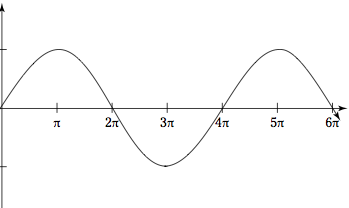π

(1/2)π

0

4π

2π

0

Explanation:

The phase displacement is the shift from the center of the graph. Since this is a sine graph and the sin(0) = 0, this is in phase.

Example Question #1 : Transformation

If this is a cosine graph, what is the phase displacement?0

(1/2)π

2π

π

4π

π

Explanation:

The phase displacement is the shift of the graph. Since cos(0) = 1, the phase shift is π because the graph is at its high point then.

Example Question #11 : Transformations

A regular pentagon is graphed in the standard (x,y) coordinate plane. Which of the following are the coordinates for the vertex P?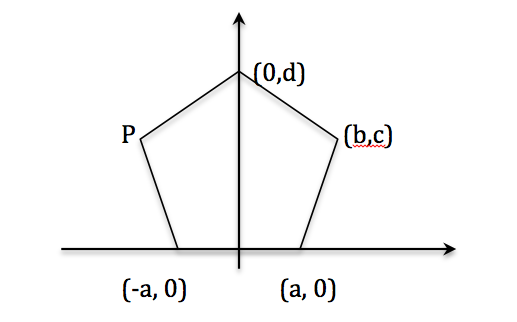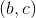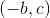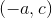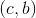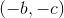Explanation:

Regular pentagons have lines of symmetry through each vertex and the center of the opposite side, meaning the y-axis forms a line of symmetry in this instance. Therefore, point P is negative b units in the x-direction, and c units in the y-direction. It is a reflection of point (b,c) across the y-axis.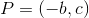Example Question #4 : How To Find Transformation For An Analytic Geometry Equation

If g(x) is a transformation of f(x) that moves the graph of f(x) four units up and three units left, what is g(x) in relation to f(x)?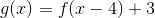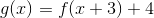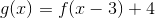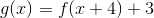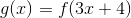Explanation:

To solve this question, you must have an understanding of standard transformations. To move a function along the x-axis in the positive direction, you must subtract the value from the operative x-value. For example, to move a function, f(x), five units to the left would be f(x+5).

To shift a function along the y-axis in the positive direction, you must add the value to the overall function. For example, to move a function, f(x), three units up would be f(x)+3.

The question asks us to move the function, f(x), left three units and up four units. f(x+3) will move the function three units to the left and f(x)+4 will move it four units up.

Together, this gives our final answer of f(x+3)+4.

Example Question #5 : How To Find Transformation For An Analytic Geometry Equation

What is the period of the function?2π

π

3π

1

4π

In this particular case we have a sine curve that starts at 0 and completes one cycle when it reaches.
Therefore, the period is